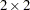PROC SURVEYFREQ

The SURVEYFREQ procedure produces one-way to n-way frequency and crosstabulation tables from sample survey data. These tables include estimates of population totals, population proportions (overall proportions, and also row and column proportions), and corresponding standard errors. Confidence limits, coefficients of variation, and design effects are also available. The procedure provides a variety of options to customize the table display.

For one-way frequency tables, PROC SURVEYFREQ provides Rao-Scott chi-square goodness-of-fit tests, which are adjusted for the sample design. You can test a null hypothesis of equal proportions for a one-way frequency table, or you can input custom null hypothesis proportions for the test. For two-way frequency tables, PROC SURVEYFREQ provides design-adjusted tests of independence, or no association, between the row and column variables. These tests include the Rao-Scott chi-square test, the Rao-Scott likelihood ratio test, the Wald chi-square test, and the Wald log-linear chi-square test.

Fortables, PROC SURVEYFREQ computes estimates and confidence limits for risks (or row proportions), the risk difference, the odds ratio, and relative risks.

PROC SURVEYFREQ uses ODS Graphics to create graphs as part of its output. Available statistical graphics include weighted frequency plots and odds ratio plots.